Skip to content
Related Articles
Congruence of Triangles
• Last Updated : 25 Jan, 2021

Pythagorean theorem is a kind of pillar of geometry. In mathematics, the Pythagorean theorem, or Pythagoras’s theorem, is a fundamental relation in Euclidean geometry among the three sides of a right triangle. Let us see the actual concept and examples of the Pythagorean theorem.

### Pythagoras Theorem Statement

If we have a triangle and the triangle has to be a right triangle, i.e. one of the three angles in a triangle has to be 90 degrees. Now, with the Pythagorean theorem, if we know two sides of a right triangle we can find out the third side.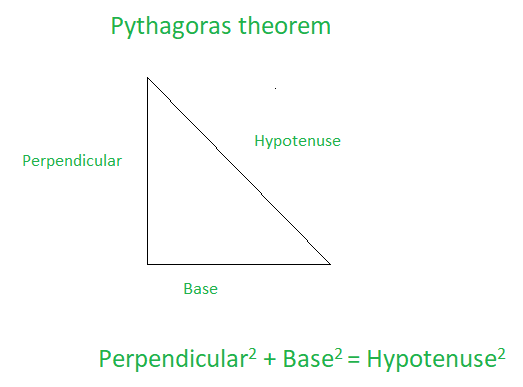The longest side of a right triangle is the side opposite to the right angle, which is the hypotenuse. Also, we can see in the above diagram, that square of two sides which is adjacent to the 90-degree angle is equal to the square of the hypotenuse.

### Pythagorean Theorem Examples

Let’s see some basic examples of the Pythagorean theorem.

Example 1: Determine the height of an equilateral triangle of side 16 units?

Solution:

For the given problem we can split the triangle into two equal parts from which we can get the hypotenuse length is 16 units. And the other leg is 8 units i.e. half of 16 units. So, let the height be x.

According to question,

x2 + 82 = 162

=> x2 = 192

=> x = √192

Taking positive value of x

=> x = 13.86 units

Hence, the required height is 13.86 units.

Example 2: A man goes 60m due west then 60m due north. Find his distance from the starting point?

Solution: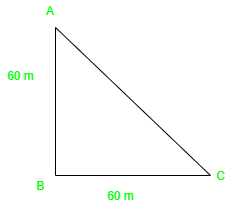According to the given question, we can draw the diagram as shown above and we have to find the distance AC.

Now, According to the Pythagoras theorem, we can get the equation as

AB2 + BC 2 = AC2

=>  AC2 = 60 + 60 2

=>  AC = 7200

=>  AC  =  √7200

=>  AC =  84.85 m ( Since, it is a distance unit. Therefore taking positive value of square root)

Hence, the required distance is 84.85 m.

### Triangle Congruence Postulates/Criteria

a. SSS (Side, Side, Side)

In the postulate of SSS, if all the three sides of a triangle are equal to the corresponding three sides of another triangle, then it satisfies the condition of SSS.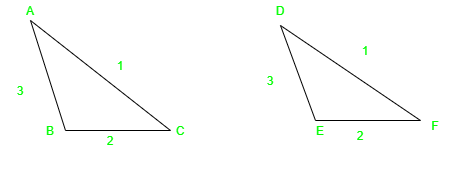In the above-given figure, we can see that, AB = DE, AC = DF and BC = EF. Hence, Δ ABC ≅ ΔDEF

b. SAS (Side, Angle, Side)

In the postulate of SAS, in comparison among two triangles, if two sides and the angle included between two sides are equal. Then it satisfies the condition of SAS.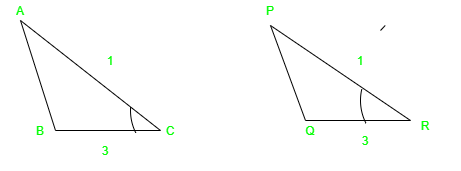In the above-given figure, we can see that, AC = PR, BC = QR, and ∠C = ∠R. Hence, ΔACB ≅ ΔPRQ.

c. ASA (Angle, Side, Angle)

Here, if we are having two triangles, where any two angles and sides included between the two angles of one triangle are equal to the angles and side of the corresponding triangle. Then the two triangles will satisfy the ASA congruency.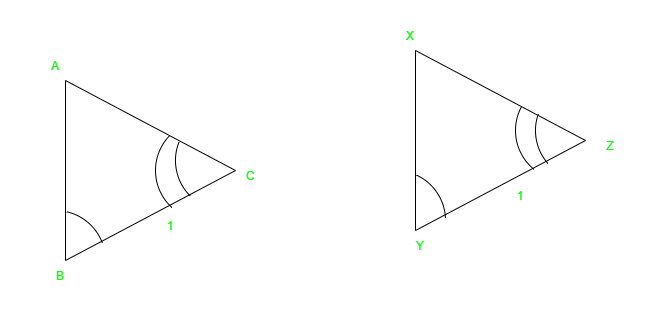In the above-given figure, we can see that, BC = YZ, ∠B = ∠Y, and ∠C = ∠Z. Hence,  ΔACB ≅  ΔXZY.

d. AAS (Angle, Angle, Side)

If any two angles of a triangle and non-included side are equal to the two angles and side of the corresponding triangle. Then it will satisfy the AAS congruency.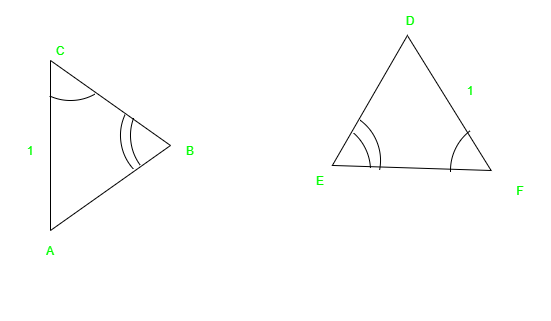From the given figure, we can say that,

AC = DF,

∠B = ∠E ,

and     ∠C = ∠F

Hence, ΔACB ≅  ΔDFE

e. RHS (Right angle- Hypotenuse- Side)

RHS criteria only get satisfied with a right-angled triangle. So, in RHS congruency, if only two sides i.e. hypotenuse and one side of a right-angled triangle are equal to the corresponding hypotenuse and side of another right-angled triangle. Then we can say that RHS satisfied.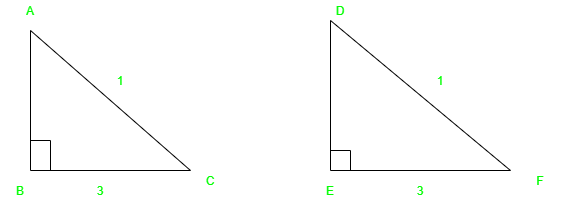From the above-given example of a right-angled triangle, we get AC = DF and BC = EF. Hence, ΔACB ≅ ΔDFE.

### Why SSA isn’t congruence postulate/criteria?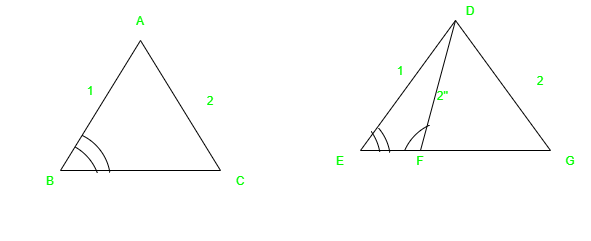Proof:

Let’s first see in Δ ABC and Δ DEG

∠B = ∠E, AB= DE and AC = DG

So, from this above condition, we cannot justify that Δ ABC ≅  ΔDEG. To understand this, let’s construct a line DF in Δ DEG as shown above, and DF is congruent to AC and DG. We have got a new triangle i.e. Δ DEF.

Now, we can see that AC is congruent to DF, ∠B = ∠E, and AC = DE. But,  Δ ABC is not congruent to ΔDEF. Because of which we can make a conclusion that SSA is not only sufficient to tell that triangles are congruent. Therefore, it is important to know the ∠A and ∠D means the angle between the two sides.

There is only one exceptional case which we have seen in the RHS postulate, on which SSA or ASS postulate will get satisfied. Henceforth, in a general or common scenario, SSA or ASS postulate will not be satisfying.

My Personal Notes arrow_drop_up